# Longest Common Subsequence

Key takeaways

• An excellent problem to learn dynamic programming approach. We are using the bottom-up approach to fill the 2-D table in a row-wise fashion. Based on this idea, we can solve a lot of other problems.
• A wonderful problem to learn space complexity optimization.

### Let’s understand the problem

Given two array strings X and Y of size m and n, write a code to find the length of the longest common sequence.

• subsequence of a string is a new string generated from the original string with some characters (can be none) deleted without changing the relative order of the remaining characters. For example, [A, C, E] is a subsequence of [A, B, C, D, E].
• common subsequence of two strings is a subsequence that is common to both strings. If there is no common subsequence, return 0. In other words, given two sequences X and Y, we say that a sequence Z is a common subsequence of X and Y if Z is a subsequence of both X and Y.
• There can be multiple possible common subsequences of the longest length, but need to just return the length of the longest common subsequence.
• Suppose m >= n.

Example 2

Input: X[ ] = [A, B, C, B, D, A, B], Y[ ]= [B, D, C, A, B, A]

Output: 4

Explanation: There are many common subsequences of X and Y. For example, the sequence [B, C, A] is a common subsequence of length 3 but it is not the longest common subsequence X and Y. Similarly, sequence [B, C, B, A], which is also common to both X and Y, has length 4. The sequence [B, C, B, A] is an LCS of X and Y, as is the sequence [B, D, A, B]. In other words, X and Y have no common subsequence of length 5 or greater.

Example 2

Input: X[] = [E, B, T, B, C, A, D, F], Y[] = [A, B, B, C, D, G, F]

Output: 5, Explanation: The longest common subsequence is [B, B, C, D, F].

### Discussed solution approaches

• A brute force approach using recursion
• An efficient approach using top-down memoization
• An efficient approach using the bottom-up approach
• Space optimized solution of the bottom-up approach
• The most efficient space optimzed solution of the bottom-up approach

### A brute force approach using recursion

Solution Idea and Steps

This is an optimization problem where solution space is very large i.e. there can be so many common subsequences of both strings, but we need to find a common subsequence with the longest length. So, the one basic idea would be to explore all the subsequences of both strings and find the longest among them. When we need to do an exhaustive search or explore all possibilities, we can try to use the idea of recursion. Think! Now the critical question would be: how do we explore all the subsequences recursively? Let's think!

Here we have one obvious choice at the start: the last characters of both strings are equal or not! If both are equal, then that common character will be part of the longest subsequences. Think! Let's assume function lcs(X, Y, m, n) returns the length of the longest subsequence.

Case 1: If the last characters of strings are equal i.e. if(X[m-1] == Y[n-1])

In this situation, the problem gets reduced to finding the longest subsequence of the remaining substring of input size m-1 and n-1.

lcs(X, Y, m, n) = 1 + lcs(X, Y, m - 1, n - 1)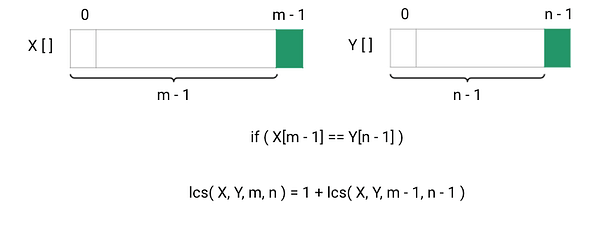Case 2: If the last characters of strings are not equal i.e. if (X[m-1] != Y[n-1])

In this situation, there are two possibilities of smaller sub-problems, and we need to find the maximum of them:

• Possibility 1: finding the longest subsequence length by excluding the last character of the string X and including the last character of the string Y i.e. lcs(X, Y, m - 1, n). Here the input size of X and is m-1 and n.
• Possibility 2: finding the longest subsequence length by including the last character of the string X and excluding the last character of the string Y i.e. lcs(X, Y, m, n - 1). Here the input size of X and Y is m and n-1.
• Now any one of both possibilities can provide the length of the longest LCS, so we need to take the maximum of both possibilities.

lcs(X, Y, m, n) = max ( lcs(X, Y, m , n - 1), lcs(X, Y, m - 1 , n) )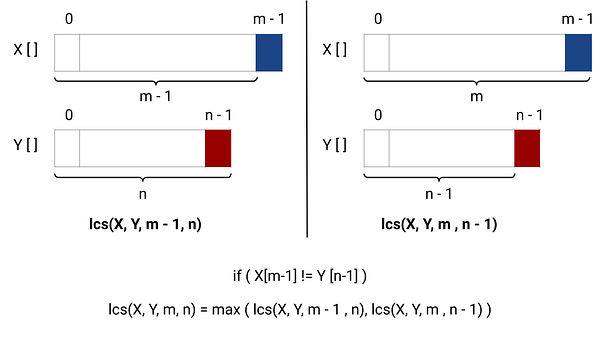Base case: recursion will terminate when any one of the string sizes gets reduced to 0 i.e. if (m == 0 || n == 0), we return 0.

Solution Pseudocode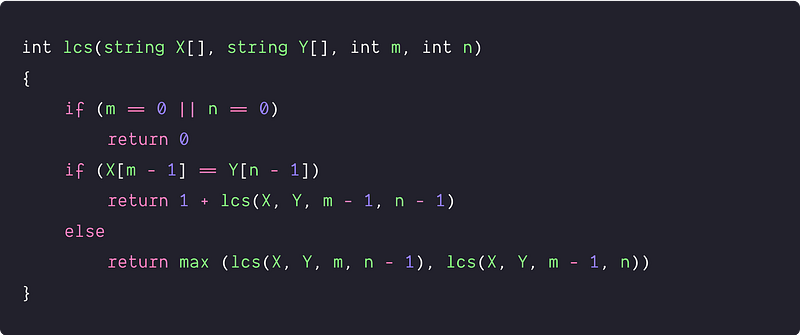Time and space complexity analysis

• We are exploring all the possible subsequences of both the string using recursion and comparing the last character. To explore all possibilities, There would be two choices for each character in a string: either it is part of the subsequence or not part of the subsequence.
• So the total number of subsequences of string X of size m = 2^m,
• The total number of subsequences of string Y of size n = 2^n
• Time complexity = O(2^m * 2^n) = O(2^(m+n)), which is exponential time. It is inefficient and impractical for long sequences.

Space complexity depends on the size of the call stack, which is equal to the height of the recursion tree. Here input parameters m and n are at max decreasing by 1, so the height of the recursion tree = max(m, n). Space complexity = max(m, n).(Think!)

Now the critical question would be: why is time complexity exponential? The answer would be simple: we have overlapping sub-problems i.e. we are solving the same sub-problems again and again during the recursion. This can be better visualized by drawing the recursion tree.

In the following diagram of the recursion tree, the subproblem of size (m-2, n-2) is coming twice. We can observe other repeating subproblems if we draw the recursion tree further.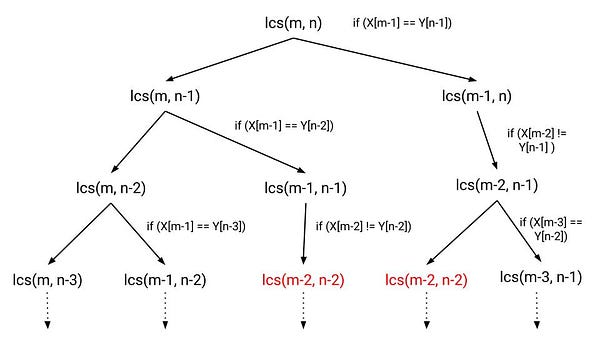### An efficient approach using top-down memoization

Solution Idea

The problem with the recursive solution is that the same subproblems get called many times. A subproblem consists of two parameters, m and n, which is at-max decreasing by 1 during each recursive call, so there are exactly (m+1)(n+1) possible subproblems. Some of these subproblems must be being solved over and over.

So one efficient idea would be: use the memoization approach of dynamic programming and store the solution of each sub-problems into an extra memory. When we encounter the same sub-problem during the recursion, we look up the extra memory and return the already calculated solution directly instead of computing it again. This could help us to reduce a lot of computation and improve the time complexity significantly. Think!

• We take extra memory of size equal to the total number of different subproblems i.e. (m+1)(n+1), where we store subproblem of input size (i, j) at the index [i+1][j+1]. (0 <= i <=m and 0 <= j <= m)
• In the LCS problem, no result is negative, so we'll use -1 as a flag to tell the algorithm that nothing has been stored yet.

Note: The dynamic programming solution is to check whenever we want to solve a subproblem, whether we've already done it before. If so, we look up the solution instead of recomputing it. Implemented in the most direct way, we just add some code to our recursive algorithm to do this look-up. This "top-down" recursive version of dynamic programming is known as "memoization".

Solution Pseudocode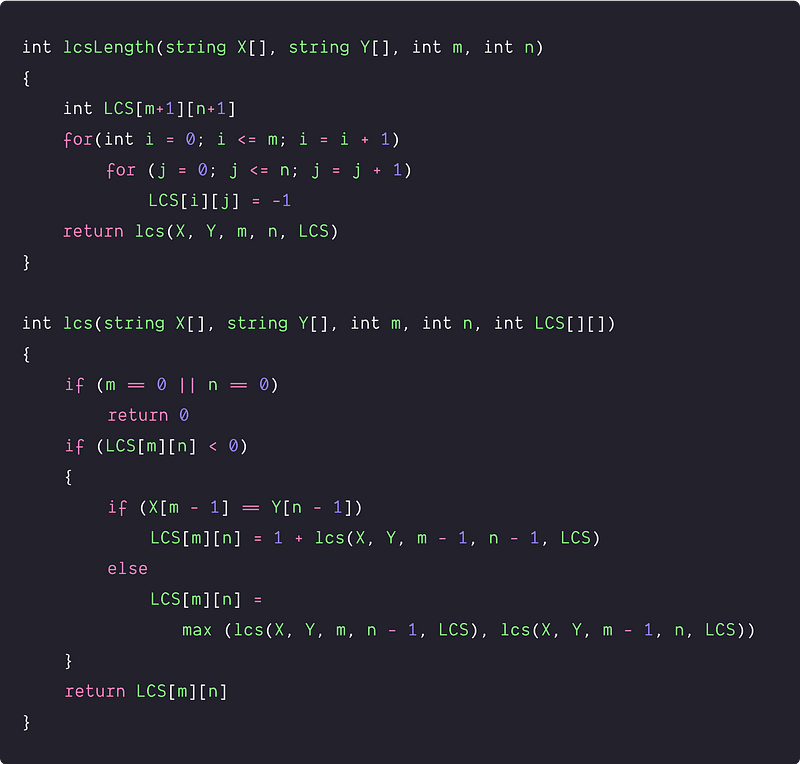Time and space complexity analysis

• We are solving each subproblem only once and each recursive call to subproblem takes constant time. We call it once from the main routine, and at most twice every time we fill in an entry of array LCS[].
• There are (m+1)(n+1) entries, so the total number of recursive calls are at most 2(m+1)(n+1)+1. So time complexity = O(m*n)
• We are using a 2-D table of size (m+1)*(n+1) to store the solutions of the subproblems. So space complexity = O(m*n)
• As usual, this is a worst-case analysis. The time might sometimes be better if not all array entries get filled out. For instance, if the two strings match exactly, we'll only fill in diagonal entries and the algorithm will be fast.

### An efficient approach using the bottom-up approach

Solution Idea and Steps

Since we have identified that it is a dynamic programming problem, we can solve it efficiently using the bottom-up approach. Our aim is to calculate the solution of the smaller problems in an iterative way and store their result in a table. But the critical question is :  how do we build the solution in a bottom-up manner? Here are the important steps:

• Table structure: The state of the smaller sub-problems depends on the input parameters m and n because at least one of them decreases after each recursive call. So we need to construct a 2-D table LCS[][] to store the solution of the sub-problems.
• Table size: The size of the 2-D table will be equal to the total number of different subproblems, which is equal to (m+1)*(n+1).
• Table initialization: Before building the solution using the iterative structure, we need to initialize the table by the smaller version of the solution i.e base case. Here m = 0 and n = 0 is the situation of the base case, so we initialize the first row and first column of the table with value 0.
• Iterative structure to fill the table: Now, we need to define the iterative structure to fill the table LCS[][] i.e. a relation by which we build the solution of the larger problem using the solution of smaller problems in a bottom-up manner. We can easily define the iterative structure by using the recursive structure of the recursive solution.

LCS[i][j] = 1 + LCS[i-1][j-1], if(X[i-1] == Y[j-1])

LCS[i][j] = max (LCS[i][j-1], LCS[i-1][j]), if(X[i-1] != Y[j-1])

• Returning final solution: After filling the table iteratively, our final solution gets stored at the bottom left corner of the 2-D table i.e. we return LCS[m][n] as an output.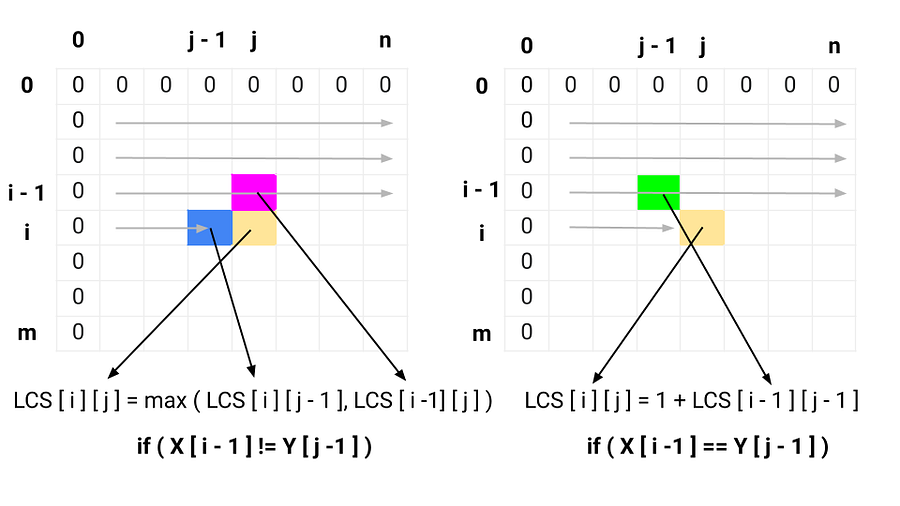Solution Pseudocode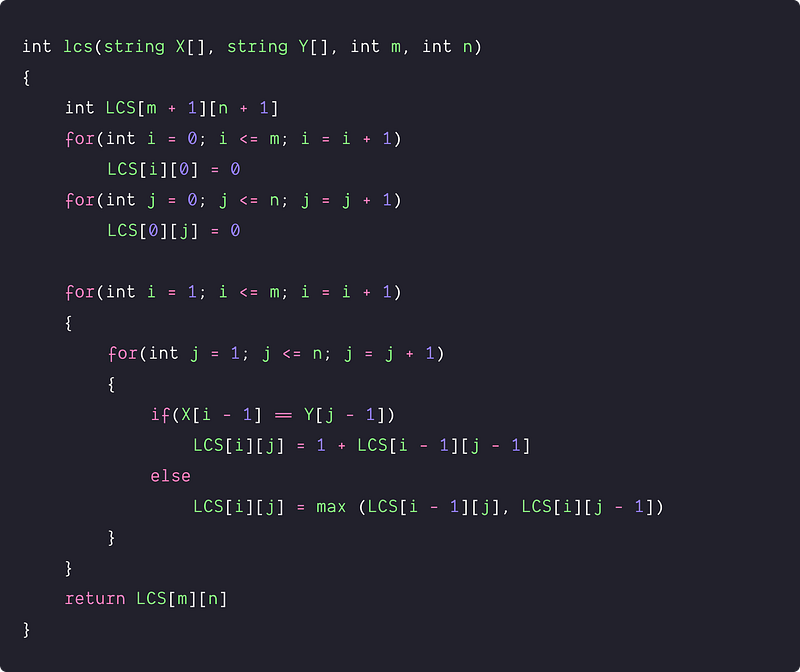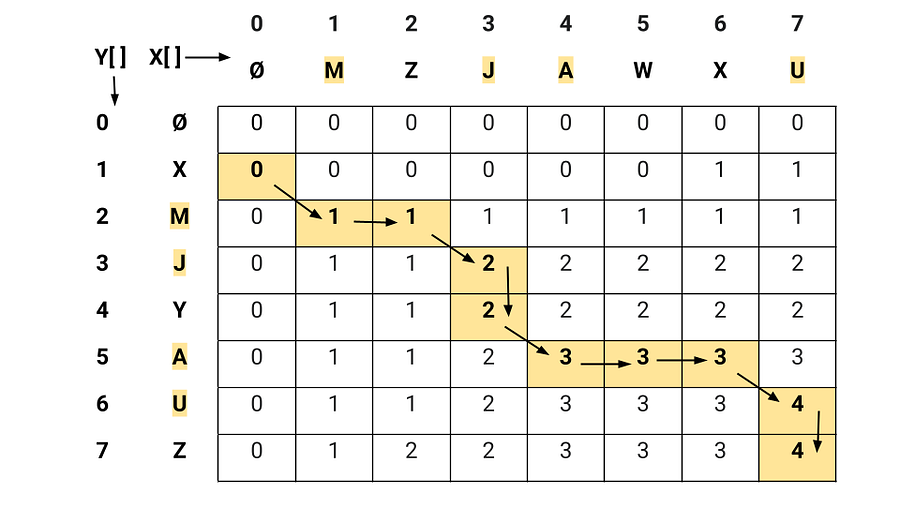Time and space complexity analysis

Time complexity = time complexity of initializing the table + time complexity of filling the table into a bottom-up manner + time complexity of returning the final solution = O(m + n) + O(mn) + O(1) = O(mn)

Space complexity = O(mn) for storing the table size (m+1)*(n+1).

### Space optimized version of the bottom-up approach

If we observe the previous 2-D solution of the problem, we are only using the adjacent indexes in the table to build the solution in a bottom-up manner. In other words, we are using LCS[i-1][j-1], LCS[i][j-1] and LCS[i-1][j] to fill the position LCS[i][j]. So there are two basic observations:

• We are filling the entries of the table in a row-wise fashion.
• To fill the current row, we only need the value stored in the previous row.

So there is no need to store all rows in our DP matrix and we can just store two rows at a time. In other words, we can use a two-row 2D array LCS[n+1] to update the values and get the output.

Solution Pseudocode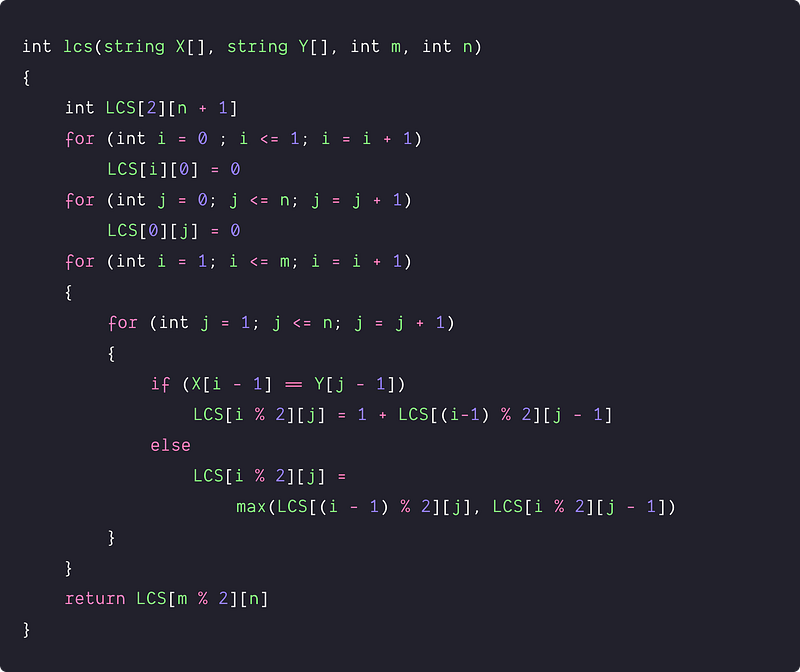Time and space complexity analysis

There is no change in time complexity in comparison to the previous approach (as we are using two nested loops similar to the previous approach). So time complexity = O(mn). Space complexity gets reduced to O(n) because the total number of rows is constant.

### Space optimized version of the bottom-up approach

Solution Idea and Steps

Now the critical question would be: can we optimize the space complexity further? The idea is: In the last approach, we are using a two-row 2-D table and storing values in a row-wise fashion using two values from the previous row. So can we store one row of data and track two previous rows values using two variables? Let's think!

1. We take a 1-D array LCS[n] to store the values of the current row.
2. Now we run nested loops similar to the last approach. Here at the ith iteration of the outer loop, we store ith row values in table LCS[n] using the inner loop. Suppose after the (i-1)th iteration of the outer loop, we have all the values of (i-1)th row stored in the table LCS[n]. Now we try to store the values of the ith row using the inner loop.

• We move forward using the inner loop from j = 1 to n to fill the table entries for the ith row. Before starting the inner loop to fill the ith row values, we initialize two variables PrevRow = 0 and preRowPrevCol = 0 to track two values of previous rows. In other words, these two variable will track values LCS[i - 1][j] and LCS[i - 1][j - 1] of 2-D matrix of the previous approach.
• At any general step of the inner loop, before storing the value at LCS[j], we update the two required values of the (i-1)th iteration in the variables PrevRow and preRowPrevCol i.e. prevRowPrevCol = prevRow, prevRow = LCS[j]. Now we have the information to store the values at the position LCS[j].
• If(X[i-1] != Y[j-1]), LCS[j] = max(LCS[j-1], prevRow). Here prevRow is the calculated value of jth index in (i-1)th iteration of the outer loop and LCS[j-1] is the calculated value of (j-1)th index in the ith iteration of the outer loop.
• If(X[i-1] == Y[j-1]), LCS[j] = prevRowPrevCol + 1. Here revRowPrevCol is the calculated value of (j-1)th index in (i-1)th iteration of the outer loop.
• Now we fill all the entries of the table LCS[n] by updating the values of both variables in a similar fashion.
3. After filling the ith row entries in the table using the inner loop, now we move to the next iteration of the outer loop to fill the (i+1)th row entries.
4. By the end of both nested loops, our final solution will get stored at the position LCS[n]. We return this value as an output.

We highly recommend visualizing this approach on paper by drawing the flow of storing values in the table by updating variables. Think!

Solution Pseudocode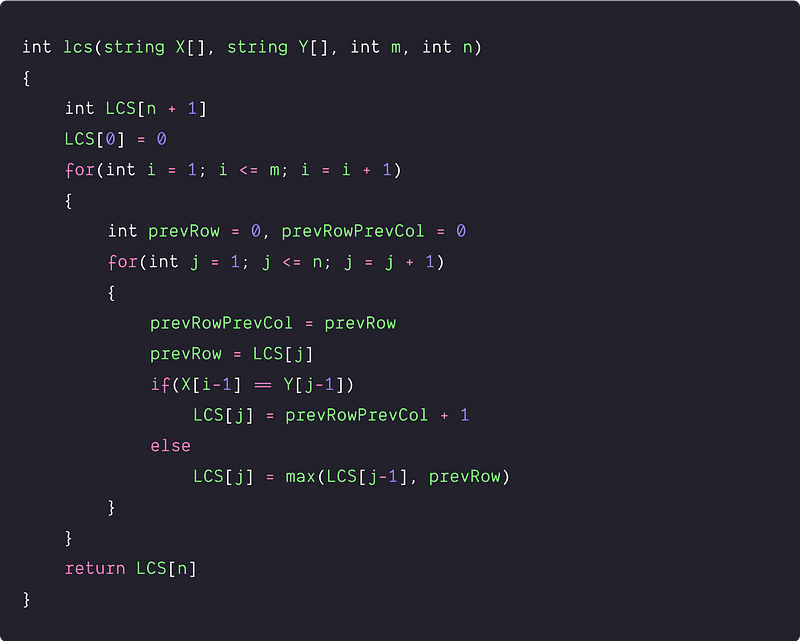Time and space complexity analysis

There is no change in time complexity as we use two nested loops similar to the previous approach. So time complexity = O(mn). Space complexity is still O(n), but it is the optimized version of the previous approach because we only use a 1-D table to store one row.

### Critical ideas to think!

• Instead of comparing the last character, can we solve this problem by comparing the first character? If yes, then how do we store solutions in the table?
• How do we modify the above approaches to also return any one of the longest subsequences? What would be the time and space complexity for it? Can we modify the above code to return the longest subsequence in lexicographically sorted order?
• In the space-optimized approach of the two-row 2-D table, can we implement it using two separate arrays of size n?
• In the last two space-optimized approaches, what would be the best version of the space complexity if n > m? How do we modify the code so that space complexity becomes O(m)?
• In the last four approaches, can we use hash tables to store solutions to the smaller sub-problems instead of using an array? If yes then which approach would be more efficient?
• What would be the worst and best scenario of the brute force recursive approach?

### An important note

Why might we want to solve the longest common subsequence problem? There are several motivating applications.

• Molecular biology. DNA sequences (genes) can be represented as sequences of four letters ACGT, corresponding to the four sub-molecules forming DNA. When biologists find a new sequence, they typically want to know what other most similar sequences are? One way of computing how similar two sequences are is to find the length of their longest common subsequence.
• File comparison. The Unix program "diff" is used to compare two different versions of the same file to determine what changes have been made to the file. It works by finding the longest common subsequence of the lines of the two files: any line in the subsequence has not been changed, so what it displays is the remaining set of lines that have changed. We should think of each file line as a single complicated character in a string in this problem.

### Suggested coding questions to practice

Edit distance problem

Shortest Common Supersequence

Wildcard Pattern Matching

Longest Palindromic Subsequence

Maximum Length of Repeated Subarray

Enjoy learning, Enjoy problem-solving!

Get well-designed application and interview centirc content on ds-algorithms, machine learning, system design and oops. Content will be delivered weekly.